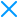# Char To Ternary Operator

Potency Medium
Resilience Low
Cost Medium
Tags: strings, literal, char, ternary, operator, obfuscation

## Description

A string is a sequence of characters used to represent text. It is one of the primitive values in JavaScript. With this transformation your single character static string values will be harder to evaluate by a human. This also generates a random output each time it is used so your code will look different after each protection.

### Code Annotation Example

```// @jscrambler define charToTernaryOperator {freq: 1, tern: [1,1]} as ctto
// @jscrambler enable ctto```

## Examples

The following example contains a simple variable assignment:

``var htmlTags = ['a', 'div', 'p'];``

After transforming, your code will look like this:

```var htmlTags = [
665.01 > 6770 ? 'Q' : (2170, 1050) !== 805 ? (9480, 813.32) >= 5227 ? 754.03 : 'a' : 7.26e+3,
'div',
(8140, 1590) === (7290, 3300) ? 'z' : (602.65, 8544) < 2637 ? (704, 4830) == 4240 ? (0xf25, 3.18e+3) : (false, 'e') : 'p'
];```

Now we have `htmlTags` first and last position transformed, but the second position remains intact because it's a string with more than one character.

If we combine this transformation with String Splitting with max potency, `div` will become three single character strings, acceptable targets of Char to Ternary Operator.

First we need to force String Splitting to be applied before Char to Ternary Operator. We achieve this through Code Annotations.

Source:

```/* @jscrambler order stringSplitting, charToTernaryOperator */
var htmlTags = ['a', 'div', 'p'];```

Becomes:

```var htmlTags = [
665.01 > 6770 ? 'Q' : (2170, 1050) !== 805 ? (9480, 813.32) >= 5227 ? 754.03 : 'a' : 7.26e+3,
((1300, 103.65) >= (458, 630.33) ? ('k', false) : 307.7 !== 568.74 ? 562 < (400.31, 4441) ? 'd' : (814.34, 120.78) : 0xffb) + ((2813, 612.26) !== 6282 ? 'i' : 377.47 < 5166 ? 266.97 != 9030 ? 120.66 : 6.37e+3 : (8.60e+3, 'G')) + (625.02 != (989.8, 6173) ? 'v' : 4940 === 654.38 ? (true, 'H') : (9825, 2740) != 35 ? ('B', 8.85e+3) : 'D'),
(8140, 1590) === (7290, 3300) ? 'z' : (602.65, 8544) < 2637 ? (704, 4830) == 4240 ? (0xf25, 3.18e+3) : (false, 'e') : 'p'
];```

Consider using Duplicate Literals Removal so that some of the literals injected by this transformation are replaced with variables declared somewhere else in the code, this results in a code more resilient to automated reversal.

Also consider using Identifiers Renaming to transform the remaining `htmlTags` from the original source code.

## Option Types

Name Required Default Value Description
freq No 0.2 Probability of applying the transformation when the node allows for the transformation to happen.
min No N/A Minimum number of times the transformation is applied to each JavaScript file. This number may still not be reached if there are not enough candidates to apply the transformation to.
max No -1 Maximum number of times the transformation is applied to each JavaScript file.
tern No [1,1] Minimum number of ternary operators. Accepts values between 1 and 3.

## Browser Compatibility

Browser Compatible Versions Tested Versions Notes
Chrome 60+ 60+
Firefox 50+ 50+
Internet Explorer 8+ 8+
Microsoft Edge 18+ 18+
Safari 9.1+ 9.1+

## API Parameters

Example:

```{
"keys": {
"accessKey": "XXXXXX",
"secretKey": "YYYYYY"
},
"applicationId": "ZZZZZZ",
"params": [
{
"name": "charToTernaryOperator",
"options": {
"tern": [
1,
1
]
}
}
]
}```